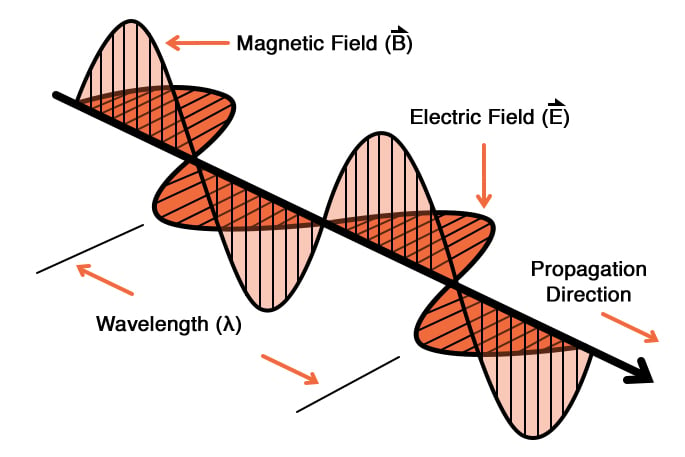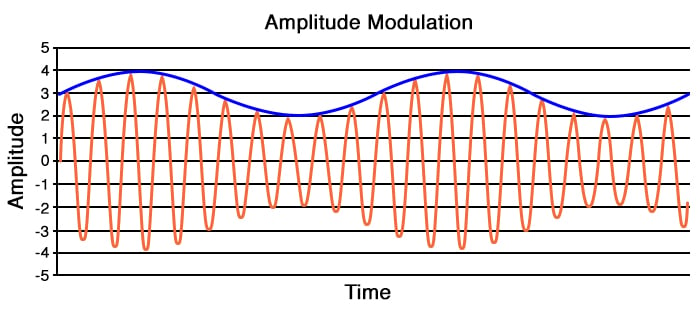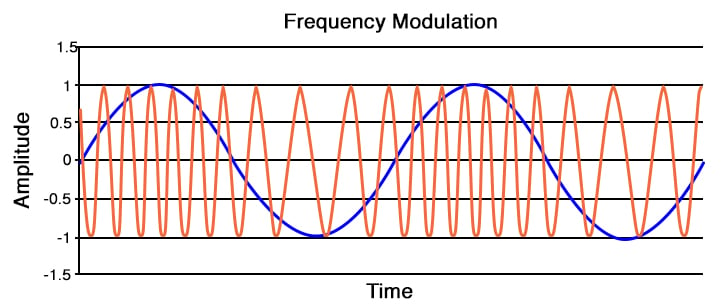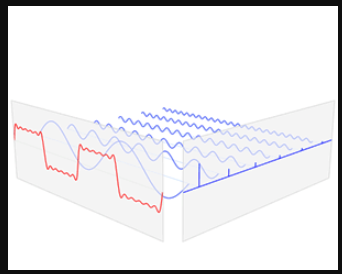# Applications of Sinusoidal Signals and Frequency-Domain Analysis

May 10, 2020 by Robert Keim
This video tutorial discusses the practical aspects of sinusoidal signals: What do they represent, and how are they used in circuits?

One reason why sinusoidal voltages and currents are so common in electrical systems is that AC facilitates the distribution of electrical power. The generators found in power plants produce sinusoidal voltage, and the amplitude of this AC voltage can be easily increased or decreased by means of transformers. In this video, though, we will focus on sinusoidal voltages and currents that transfer information rather than electrical power.

## Sinusoids in Nature

The importance of sinusoidal electrical signals is closely related to the fact that natural phenomena have sinusoidal characteristics. Sound and water waves, for example, can be represented as sinusoids, and simple harmonic motion—such as that of a pendulum or a weight attached to a spring—results in a sinusoidal relationship between position and time. Also, electromagnetic radiation propagates as a wave composed of sinusoidally oscillating electric and magnetic fields.##### This diagram conveys the way in which electromagnetic radiation propagates.

Electric circuits and systems don’t exist in isolation. Rather, they are a means of controlling mechanical components, monitoring physical processes, generating or receiving electromagnetic signals, and analyzing environmental variations. Sinusoidal relationships are present in the world that surrounds an electronic device, and consequently many electronic devices incorporate sinusoidal signals.

## Audio

The recording and reproduction of sound is one of the most influential and well-known applications of electronic technology, and sinusoidal signals figure prominently in the work of electrical engineers who design audio systems.

Microphones generate sinusoidal voltage signals that correspond to variations in air pressure; these are delivered to amplifiers, which produce sinusoidal signals that can be analyzed, modified, or stored for later use; power amplifiers convert sinusoidal voltages into signals that can drive a speaker; and speakers convert sinusoidal signals back into air-pressure variations. We can also synthesize sound by using electronic oscillators to generate sinusoidal voltages whose frequencies correspond, for example, to the notes of a musical scale.

## Wireless Communication

The ability to wirelessly transfer sound, video, and data has transformed human society. Radio systems make this type of communication possible by generating sinusoidal signals that, with the help of an amplifier and an antenna, produce electromagnetic radiation that can travel long distances and pass through solid objects.

Special modulation techniques are used to modify sinusoidal signals such that they can carry information from a transmitter to a receiver. In the previous video we discussed three characteristics that distinguish one sinusoid from another: amplitude, frequency, and phase. Radio-frequency electrical engineers use these same parameters as modulation variables; changes in these variables can be transferred as electromagnetic radiation, recovered by an electronic receiver, and interpreted as an information-bearing signal. The following diagrams will help you to visualize the two common types of analog modulation:## Frequency-Domain Analysis

Some very interesting applications of sinusoids take place in circuits that don’t have sinusoidal signals—or at least the signals do not appear to be sinusoidal.

It turns out that electrical signals can be analyzed as though they are produced through the addition of multiple sine waves with different frequencies and amplitudes. This concept is based on the Fourier transform, which is a mathematical process that converts a normal signal into a series of sinusoids that represent the signal’s frequency content. When we analyze a signal as an amplitude that varies with respect to time, we are working in the time domain. If, on the other hand, we analyze a signal as a collection of frequencies, we are working in the frequency domain.

The diagram below gives you an idea of how multiple sine waves can combine into a signal that does not have a sinusoidal shape.## Conclusion

This tutorial completes our introduction to electrical signals. In the next chapter, we’ll explore the characteristics and functionality of the most important electronic components.